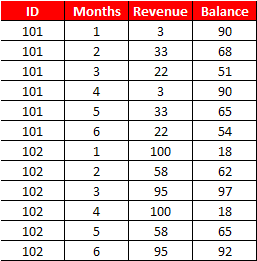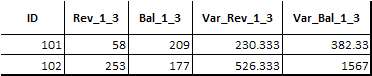# Intermediate Proc SQL Tutorial

Example 1 : Count Cases Where Condition is TRUE

The input data is shown below. Suppose you are asked to calculate the number of Ys and Ns of column Z by column X.Intermediate SQL Tutorial

data xyz;
input x y\$ z\$;
cards;
1 23 Y
1 24 N
1 25 Y
2 21 Y
2 22 Y
3 25 N
3 36 Y
;
run;
proc sql noprint;
create table tt as
select x,
sum(case when z= "Y" then 1 else 0 end) as z_Y,
sum(case when z= "N" then 1 else 0 end) as z_N
from xyz
group by x;
quit;

Example 2 : Creating trend variablesSample Dataset
data example1;
input ID Months Revenue Balance;
cards;
101 1 3 90
101 2 33 68
101 3 22 51
101 4 3 90
101 5 33 65
101 6 22 54
102 1 100 18
102 2 58 62
102 3 95 97
102 4 100 18
102 5 58 65
102 6 95 92
;
Task : Calculate total revenue and total balance accumulated in the first 3 months and how much the data spreads in the first 3 months time period.
proc sql noprint;
create table output1 as
select ID,
sum(case when 1 <= Months <= 3 then Revenue else . end) as Rev_1_3,
sum(case when 1 <= Months <= 3 then Balance else . end) as Bal_1_3,
var(case when 1 <= Months <= 3 then Revenue else . end) as Var_Rev_1_3,
var(case when 1 <= Months <= 3 then Balance else . end) as Var_Bal_1_3
from example1
group by ID;
quit;

Output :Output
Detailed Code for Multiple Variables

Example 3 : Check the Status Flag between the start date and end date

DATA dates;
INPUT ID Period : date9. Status \$;
FORMAT Period date9.;
CARDS;
1 13may2000 Y
1 17oct1999 Y
1 03feb2001 N
1 28feb2001 N
2 10nov2000 Y
2 25apr2001 N
3 03jun1997 Y
3 14jan2001 Y
3 24oct1998 N
3 27aug2000 N
;
RUN;
proc sql;
select a.ID , a.Period as Start_Date, b.Period as End_Date,
a.Status as Status_Start, b.Status as Status_End
from dates a left join
(select * from dates group by ID having Period = max(Period)) b
on a.ID = b.ID
group by a.ID
having a.Period = min(a.Period);
quit;

Example 4 : Calculate Percentage Change between the first and last row
data temp;
input ID time \$ x1-x3;
cards;
1 Y1 85 85 86
1 Y2 80 79 70
1 Y3 78 77 87
2 Y1 79 79 79
2 Y2 83 83 85
;
run;
data temp2;
set temp;
retain Serial 0;
If first.ID then Serial = 1;
else Serial = Serial + 1;
by ID;
run;
proc sql noprint;
create table t2 as
select a.ID, (a.x1-b.x1)/b.x1 *100 as change
from temp2 a left join
(select ID, x1 from temp2
group by ID
having serial = min(serial)) b
on a.ID = b.ID
group by a.ID
having serial = max(serial);
quit;

Example 5 : Extract First and Last Observation within a Group

PROC SQL : Alternative to First. Statement
proc sql;
create table output2 (drop=n) as
select *, monotonic() as n
from example1
group by ID
having min(n) = n;
quit;
PROC SQL : Alternative to Last. Statement
proc sql;
create table output2 (drop=n) as
select *, monotonic() as n
from example1
group by ID
having max(n) = n;
quit;
Example 6 : Self Join
Suppose you have data for employees. It comprises of employees' name, ID and manager ID. You need to find out manager name.Proc SQL : Self Join
data example2;
input Name \$ ID ManagerID;
cards;
Smith 123 456
Robert 456 .
William 222 456
Daniel 777 222
Cook 383 222
;
run;
SQL Query : Self Join
proc sql;
create table want as
select a.*, b.Name as Manager
from example2 as a left join example2 as b
on a.managerid = b.id;
quit;
Example 7 : Capping Extreme Values
Suppose you need to cap values of a column.

data have1;
input x y z;
cards;
101  1 10
102  2 20
103  3 45
104  1 23
105  2 42
106  3 46
107  1 61
109  2 22
110  3 28
111  1 30
112  2 32
113  3 39
;
run;
proc sql noprint;
create table output2 (drop=z rename= (z1=z))  as
select *, case when z >=  40 then 40 else z
end as z1 from have1;
quit;
First cap values of column z and then sum all the z values by column y.
proc sql;
select sum(z1) as OutCol
from (select *, case when z >=  40 then 40 else z
end as z1 from have1)
group by y;
quit;

Example 8 : Select All Excluding 1 Variable
proc sql;
create table want (drop=x) as
select a.*,b.*
from dat1 a, dat2 (rename=(id=x)) b
where a.id = b.x;
quit;
Example 9 : Sub Query - ANY and ALL Operators

1. ANY operator selects values that pass the comparison test with any of the values that are returned by the sub-query.

2. ALL operator selects values that pass the comparison test with all of the values that are returned by the sub-query.
data jansale;
input sale id;
cards;
100 1
105 2
108 3
110 4
;
run;
data febsale;
input sale id;
cards;
120 1
105 2
118 3
117 4
;
run;
proc sql;
select *
from jansale
where sale < all (select sale from febsale);
quit;
proc sql;
select *
from jansale
where sale < any (select sale from febsale);
quit;Share
Related PostsDeepanshu founded ListenData with a simple objective - Make analytics easy to understand and follow. He has over 10 years of experience in data science. During his tenure, he has worked with global clients in various domains like Banking, Insurance, Private Equity, Telecom and Human Resource.

4 Responses to "Intermediate Proc SQL Tutorial"
1.This comment has been removed by the author.

2.This comment has been removed by the author.

3.hello , in code 2 , what does revenue else . end means ? Also i am unable to download detaled code file , as it is password protected .can you please share the password.

4.